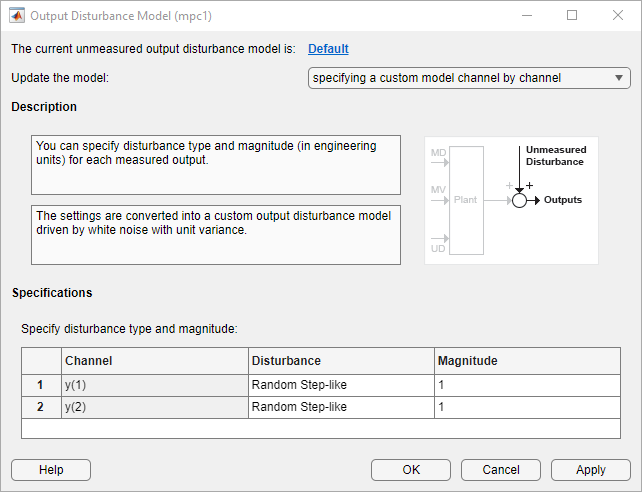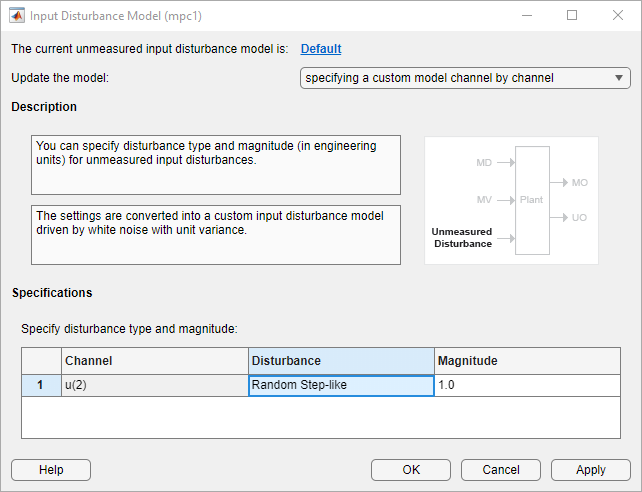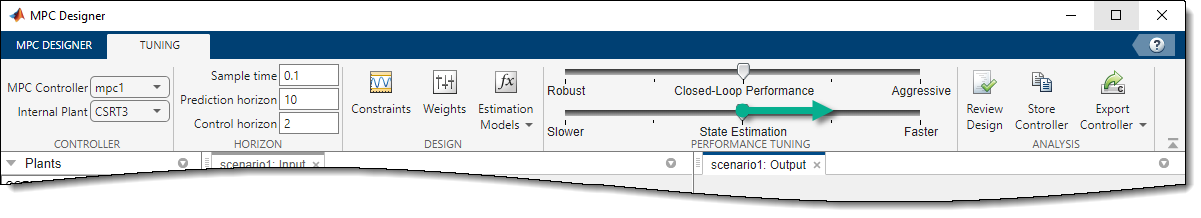A model predictive controller requires the following to reject unknown disturbances effectively:

• Application-specific disturbance models

• Measurement feedback to update the controller state estimates

You can modify input and output disturbance models, and the measurement noise model using the MPC Designer app and at the command line. You can then adjust controller tuning weights to improve disturbance rejection.

Overview

MPC attempts to predict how known and unknown events affect the plant output variables (OVs). Known events are changes in the measured plant input variables (MV and MD inputs). The plant model of the controller predicts the impact of these events, and such predictions can be quite accurate. For more information, see MPC Prediction Models.

The impacts of unknown events appear as errors in the predictions of known events. These errors are, by definition, impossible to predict accurately. However, an ability to anticipate trends can improve disturbance rejection. For example, suppose that the control system has been operating at a near-steady condition with all measured OVs near their predicted values. There are no known events, but one or more of these OVs suddenly deviates from its prediction. The controller disturbance and measurement noise models allow you to provide guidance on how to handle such errors.

Output Disturbance Model

Suppose that your plant model includes no unmeasured disturbance inputs. The MPC controller then models unknown events using an output disturbance model. As shown in MPC Prediction Models, the output disturbance model is independent of the plant, and its output adds directly to that of the plant model.

Using MPC Designer, you can specify the type of noise that is expected to affect each plant OV. In the app, on the Tuning tab, in the Design section, click Estimation Models > Output Disturbance Model. In the Output Disturbance Model dialog box, in the Update the model drop-down list, select specifying a custom model channel by channel.In the Specifications section, in the Disturbance column, select one of the following disturbance models for each output:

• White Noise — Prediction errors are due to random zero-mean white noise. This option implies that the impact of the disturbance is short-lived, and therefore requires a modest, short-term controller response.

• Random Step-like — Prediction errors are due to a random step-like disturbance, which lasts indefinitely, maintaining a roughly constant magnitude. Such a disturbance requires a more aggressive, sustained controller response.

• Random Ramp-like — Prediction errors are due to a random ramp-like disturbance, which lasts indefinitely and tends to grow with time. Such a disturbance requires an even more aggressive controller response.

Model Predictive Control Toolbox™ software represents each disturbance type as a model in which white noise, with zero mean and unit variance, enters a SISO dynamic system consisting of one of the following:

• A static gain — For a white noise disturbance

• An integrator in series with a static gain — For a step-like disturbance

• Two integrators in series with a static gain — For a ramp-like disturbance

You can also specify the white noise input Magnitude for each disturbance model, overriding the assumption of unit variance. As you increase the noise magnitude, the controller responds more aggressively to a given prediction error. The specified noise magnitude corresponds to the static gain in the SISO model for each type of noise.

You can also view or modify the output disturbance model from the command line using getoutdist and setoutdist respectively.

Measurement Noise Model

MPC also attempts to distinguish disturbances, which require a controller response, from measurement noise, which the controller should ignore. Using MPC Designer, you can specify the expected measurement noise magnitude and character. In the app, on the Tuning tab, in the Design section, click Estimation Models > Measurement Noise Model. In the Model Noise Model dialog box, in the Update the model drop-down list, select specifying a custom model channel by channel.

In the Specifications section, in the Disturbance column, select a noise model for each measured output channel. The noise options are the same as the output disturbance model options.White Noise is the default option and, in nearly all applications, should provide adequate performance.

When you include a measurement noise model, the controller considers each prediction error to be a combination of disturbance and noise effects. Qualitatively, as you increase the specified noise Magnitude, the controller attributes a larger fraction of each prediction error to noise, and it responds less aggressively. Ultimately, the controller stops responding to prediction errors and only changes its MVs when you change the OV or MV reference signals.

Input Disturbance Model

When your plant model includes unmeasured disturbance (UD) inputs, the controller can use an input disturbance model in addition to the standard output disturbance model. The former provides more flexibility and is generated automatically by default. If the chosen input disturbance model does not appear to allow complete elimination of sustained disturbances, an output disturbance model is also added by default.

As shown in MPC Prediction Models, the input disturbance model consists of one or more white noise signals, with unit variance and zero mean, entering a dynamic system. The outputs of this system are the UD inputs to the plant model. In contrast to the output disturbance model, input disturbances affect the plant outputs in a more complex way as they pass through the plant model dynamics.

As with the output disturbance model, you can use MPC Designer to specify the type of disturbance you expect for each UD input. In the app, on the Tuning tab, in the Design section, click Estimation Models > Input Disturbance Model. In the Input Disturbance Model dialog box, in the Update the model drop-down list, select specifying a custom model channel by channel.In the Specifications section, in the Disturbance column, select a noise model for each measured output channel. The input disturbance model options are the same as the output disturbance model options.

A common approach is to model unknown events as disturbances adding to the plant MVs. These disturbances, termed load disturbances in many texts, are realistic in that some unknown events are failures to set the MVs to the values requested by the controller. You can create a load disturbance model as follows:

1. Begin with an LTI plant model, Plant, in which all inputs are known (MVs and MDs).

2. Obtain the state-space matrices of Plant. For example:

[A,B,C,D] = ssdata(Plant);
3. Suppose that there are nu MVs. Set Bu = columns of B corresponding to the MVs. Also, set Du = columns of D corresponding to the MVs.

4. Redefine the plant model to include nu additional inputs. For example:

Plant.B = [B Bu];
Plant.D = [D Du]);
5. To indicate that the new inputs are unmeasured disturbances, use setmpcsignals, or set the Plant.InputGroup property.

This procedure adds load disturbance inputs without increasing the number of states in the plant model.

By default, given a plant model containing load disturbances, the Model Predictive Control Toolbox software creates an input disturbance model that generates nym step-like load disturbances. If nym > nu, it also creates an output disturbance model with integrated white noise adding to (nymnu) measured outputs. If nym < nu, the last (nunym) load disturbances are zero by default. You can modify these defaults using MPC Designer.

You can also view or modify the input disturbance model from the command line using getindist and setindist respectively.

Restrictions

As discussed in Controller State Estimation, the plant, disturbance, and noise models combine to form a state observer, which must be detectable using the measured plant outputs. If not, the software displays a command-window error message when you attempt to use the controller.

This limitation restricts the form of the disturbance and noise models. If any models are defined as anything other than white noise with a static gain, their model states must be detectable. For example, an integrated white noise disturbance adding to an unmeasured OV would be undetectable. MPC Designer prevents you from choosing such a model. Similarly, the number of measured disturbances, nym, limits the number of step-like UD inputs from an input disturbance model.

By default, the Model Predictive Control Toolbox software creates detectable models. If you modify the default assumptions (or change nym) and encounter a detectability error, you can revert to the default case.

Disturbance Rejection Tuning

During the design process, you can tune the disturbance rejection properties of the controller.

1. Before any controller tuning, define scale factors for each plant input and output variable (see Specify Scale Factors). In the context of disturbance and noise modeling, this makes the default assumption of unit-variance white noise inputs more likely to yield good performance.

2. Initially, keep the disturbance models in their default configuration.

3. After tuning the cost function weights (see Tune Weights), test your controller response to an unmeasured disturbance input other than a step disturbance at the plant output. Specifically, if your plant model includes UD inputs, simulate a disturbance using one or more of these. Otherwise, simulate one or more load disturbances, that is, a step disturbance added to a designated MV. Both MPC Designer and the sim command support such simulations.

4. If the response in the simulations is too sluggish, try one or more of the following to produce more aggressive disturbance rejection:

• Increase all disturbance model gains by a multiplicative factor. In MPC Designer, do this by increasing the magnitude of each disturbance. If this helps but is insufficient, increase the magnitude further.

• Decrease the measurement noise gains by a multiplicative factor. In MPC Designer, do this by increasing the measurement noise magnitude. If this helps but is insufficient, increase the magnitude further.

• In MPC Designer, in the Tuning tab, drag the State Estimation slider to the right. Moving towards Faster state estimation simultaneously increases the gains for disturbance models and decreases the gains for noise models.If this helps but is insufficient, drag the slider further to the right.

• Change one or more disturbances to model that requires a more aggressive controller response. For example, change the model from white noise disturbance to a step-like disturbance.

Note

Changing the disturbances in this way adds states to disturbance model, which can cause violations of the state observer detectability restriction.

5. If the response is too aggressive, and in particular, if the controller is not robust when its prediction of known events is inaccurate, try reversing the previous adjustments.